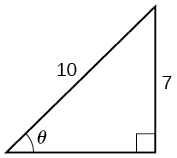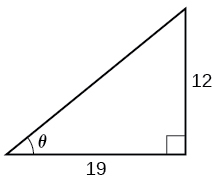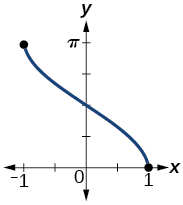# 8.3 Inverse trigonometric functions  (Page 7/15)

 Page 7 / 15

Discuss why this statement is incorrect: $\text{\hspace{0.17em}}\mathrm{arccos}\left(\mathrm{cos}\text{\hspace{0.17em}}x\right)=x\text{\hspace{0.17em}}$ for all $\text{\hspace{0.17em}}x.$

Determine whether the following statement is true or false and explain your answer: $\mathrm{arccos}\left(-x\right)=\pi -\mathrm{arccos}\text{\hspace{0.17em}}x.$

True . The angle, $\text{\hspace{0.17em}}{\theta }_{1}\text{\hspace{0.17em}}$ that equals $\text{\hspace{0.17em}}\mathrm{arccos}\left(-x\right)\text{\hspace{0.17em}}$ , $\text{\hspace{0.17em}}x>0\text{\hspace{0.17em}}$ , will be a second quadrant angle with reference angle, $\text{\hspace{0.17em}}{\theta }_{2}\text{\hspace{0.17em}}$ , where $\text{\hspace{0.17em}}{\theta }_{2}\text{\hspace{0.17em}}$ equals $\text{\hspace{0.17em}}\mathrm{arccos}x$ , $x>0\text{\hspace{0.17em}}$ . Since $\text{\hspace{0.17em}}{\theta }_{2}\text{\hspace{0.17em}}$ is the reference angle for $\text{\hspace{0.17em}}{\theta }_{1}$ , ${\theta }_{2}=\pi -{\theta }_{1}\text{\hspace{0.17em}}$ and $\text{\hspace{0.17em}}\mathrm{arccos}\left(-x\right)\text{\hspace{0.17em}}$ = $\text{\hspace{0.17em}}\pi -\mathrm{arccos}x$ -

## Algebraic

For the following exercises, evaluate the expressions.

${\mathrm{sin}}^{-1}\left(\frac{\sqrt{2}}{2}\right)$

${\mathrm{sin}}^{-1}\left(-\frac{1}{2}\right)$

$-\frac{\pi }{6}$

${\mathrm{cos}}^{-1}\left(\frac{1}{2}\right)$

${\mathrm{cos}}^{-1}\left(-\frac{\sqrt{2}}{2}\right)$

$\frac{3\pi }{4}$

${\mathrm{tan}}^{-1}\left(1\right)$

${\mathrm{tan}}^{-1}\left(-\sqrt{3}\right)$

$-\frac{\pi }{3}$

${\mathrm{tan}}^{-1}\left(-1\right)$

${\mathrm{tan}}^{-1}\left(\sqrt{3}\right)$

$\frac{\pi }{3}$

${\mathrm{tan}}^{-1}\left(\frac{-1}{\sqrt{3}}\right)$

For the following exercises, use a calculator to evaluate each expression. Express answers to the nearest hundredth.

${\mathrm{cos}}^{-1}\left(-0.4\right)$

1.98

$\mathrm{arcsin}\left(0.23\right)$

$\mathrm{arccos}\left(\frac{3}{5}\right)$

0.93

${\mathrm{cos}}^{-1}\left(0.8\right)$

${\mathrm{tan}}^{-1}\left(6\right)$

1.41

For the following exercises, find the angle $\text{\hspace{0.17em}}\theta \text{\hspace{0.17em}}$ in the given right triangle. Round answers to the nearest hundredth.For the following exercises, find the exact value, if possible, without a calculator. If it is not possible, explain why.

${\mathrm{sin}}^{-1}\left(\mathrm{cos}\left(\pi \right)\right)$

${\mathrm{tan}}^{-1}\left(\mathrm{sin}\left(\pi \right)\right)$

0

${\mathrm{cos}}^{-1}\left(\mathrm{sin}\left(\frac{\pi }{3}\right)\right)$

${\mathrm{tan}}^{-1}\left(\mathrm{sin}\left(\frac{\pi }{3}\right)\right)$

0.71

${\mathrm{sin}}^{-1}\left(\mathrm{cos}\left(\frac{-\pi }{2}\right)\right)$

${\mathrm{tan}}^{-1}\left(\mathrm{sin}\left(\frac{4\pi }{3}\right)\right)$

-0.71

${\mathrm{sin}}^{-1}\left(\mathrm{sin}\left(\frac{5\pi }{6}\right)\right)$

${\mathrm{tan}}^{-1}\left(\mathrm{sin}\left(\frac{-5\pi }{2}\right)\right)$

$-\frac{\pi }{4}$

$\mathrm{cos}\left({\mathrm{sin}}^{-1}\left(\frac{4}{5}\right)\right)$

$\mathrm{sin}\left({\mathrm{cos}}^{-1}\left(\frac{3}{5}\right)\right)$

0.8

$\mathrm{sin}\left({\mathrm{tan}}^{-1}\left(\frac{4}{3}\right)\right)$

$\mathrm{cos}\left({\mathrm{tan}}^{-1}\left(\frac{12}{5}\right)\right)$

$\frac{5}{13}$

$\mathrm{cos}\left({\mathrm{sin}}^{-1}\left(\frac{1}{2}\right)\right)$

For the following exercises, find the exact value of the expression in terms of $\text{\hspace{0.17em}}x\text{\hspace{0.17em}}$ with the help of a reference triangle.

$\mathrm{tan}\left({\mathrm{sin}}^{-1}\left(x-1\right)\right)$

$\frac{x-1}{\sqrt{-{x}^{2}+2x}}$

$\mathrm{sin}\left({\mathrm{cos}}^{-1}\left(1-x\right)\right)$

$\mathrm{cos}\left({\mathrm{sin}}^{-1}\left(\frac{1}{x}\right)\right)$

$\frac{\sqrt{{x}^{2}-1}}{x}$

$\mathrm{cos}\left({\mathrm{tan}}^{-1}\left(3x-1\right)\right)$

$\mathrm{tan}\left({\mathrm{sin}}^{-1}\left(x+\frac{1}{2}\right)\right)$

$\frac{x+0.5}{\sqrt{-{x}^{2}-x+\frac{3}{4}}}$

## Extensions

For the following exercises, evaluate the expression without using a calculator. Give the exact value.

$\frac{{\mathrm{sin}}^{-1}\left(\frac{1}{2}\right)-{\mathrm{cos}}^{-1}\left(\frac{\sqrt{2}}{2}\right)+{\mathrm{sin}}^{-1}\left(\frac{\sqrt{3}}{2}\right)-{\mathrm{cos}}^{-1}\left(1\right)}{{\mathrm{cos}}^{-1}\left(\frac{\sqrt{3}}{2}\right)-{\mathrm{sin}}^{-1}\left(\frac{\sqrt{2}}{2}\right)+{\mathrm{cos}}^{-1}\left(\frac{1}{2}\right)-{\mathrm{sin}}^{-1}\left(0\right)}$

For the following exercises, find the function if $\text{\hspace{0.17em}}\mathrm{sin}\text{\hspace{0.17em}}t=\frac{x}{x+1}.$

$\mathrm{cos}\text{\hspace{0.17em}}t$

$\frac{\sqrt{2x+1}}{x+1}$

$\mathrm{sec}\text{\hspace{0.17em}}t$

$\mathrm{cot}\text{\hspace{0.17em}}t$

$\frac{\sqrt{2x+1}}{x}$

$\mathrm{cos}\left({\mathrm{sin}}^{-1}\left(\frac{x}{x+1}\right)\right)$

${\mathrm{tan}}^{-1}\left(\frac{x}{\sqrt{2x+1}}\right)$

$t$

## Graphical

Graph $\text{\hspace{0.17em}}y={\mathrm{sin}}^{-1}x\text{\hspace{0.17em}}$ and state the domain and range of the function.

Graph $\text{\hspace{0.17em}}y=\mathrm{arccos}\text{\hspace{0.17em}}x\text{\hspace{0.17em}}$ and state the domain and range of the function.domain $\text{\hspace{0.17em}}\left[-1,1\right];\text{\hspace{0.17em}}$ range $\text{\hspace{0.17em}}\left[0,\pi \right]\text{\hspace{0.17em}}$

Graph one cycle of $\text{\hspace{0.17em}}y={\mathrm{tan}}^{-1}x\text{\hspace{0.17em}}$ and state the domain and range of the function.

For what value of $\text{\hspace{0.17em}}x\text{\hspace{0.17em}}$ does $\text{\hspace{0.17em}}\mathrm{sin}\text{\hspace{0.17em}}x={\mathrm{sin}}^{-1}x?\text{\hspace{0.17em}}$ Use a graphing calculator to approximate the answer.

approximately $\text{\hspace{0.17em}}x=0.00\text{\hspace{0.17em}}$

For what value of $\text{\hspace{0.17em}}x\text{\hspace{0.17em}}$ does $\text{\hspace{0.17em}}\mathrm{cos}\text{\hspace{0.17em}}x={\mathrm{cos}}^{-1}x?\text{\hspace{0.17em}}$ Use a graphing calculator to approximate the answer.

## Real-world applications

Suppose a 13-foot ladder is leaning against a building, reaching to the bottom of a second-ﬂoor window 12 feet above the ground. What angle, in radians, does the ladder make with the building?

Suppose you drive 0.6 miles on a road so that the vertical distance changes from 0 to 150 feet. What is the angle of elevation of the road?

An isosceles triangle has two congruent sides of length 9 inches. The remaining side has a length of 8 inches. Find the angle that a side of 9 inches makes with the 8-inch side.

Without using a calculator, approximate the value of $\text{\hspace{0.17em}}\mathrm{arctan}\left(10,000\right).\text{\hspace{0.17em}}$ Explain why your answer is reasonable.

A truss for the roof of a house is constructed from two identical right triangles. Each has a base of 12 feet and height of 4 feet. Find the measure of the acute angle adjacent to the 4-foot side.

A laser rangefinder is locked on a comet approaching Earth. The distance g(x), in kilometers, of the comet after x days, for x in the interval 0 to 30 days, is given by g(x)=250,000csc(π30x). Graph g(x) on the interval [0, 35]. Evaluate g(5)  and interpret the information. What is the minimum distance between the comet and Earth? When does this occur? To which constant in the equation does this correspond? Find and discuss the meaning of any vertical asymptotes.
The sequence is {1,-1,1-1.....} has
how can we solve this problem
Sin(A+B) = sinBcosA+cosBsinA
Prove it
Eseka
Eseka
hi
Joel
June needs 45 gallons of punch. 2 different coolers. Bigger cooler is 5 times as large as smaller cooler. How many gallons in each cooler?
7.5 and 37.5
Nando
find the sum of 28th term of the AP 3+10+17+---------
I think you should say "28 terms" instead of "28th term"
Vedant
the 28th term is 175
Nando
192
Kenneth
if sequence sn is a such that sn>0 for all n and lim sn=0than prove that lim (s1 s2............ sn) ke hole power n =n
write down the polynomial function with root 1/3,2,-3 with solution
if A and B are subspaces of V prove that (A+B)/B=A/(A-B)
write down the value of each of the following in surd form a)cos(-65°) b)sin(-180°)c)tan(225°)d)tan(135°)
Prove that (sinA/1-cosA - 1-cosA/sinA) (cosA/1-sinA - 1-sinA/cosA) = 4
what is the answer to dividing negative index
In a triangle ABC prove that. (b+c)cosA+(c+a)cosB+(a+b)cisC=a+b+c.
give me the waec 2019 questionsByByByByByByBy